Related Articles
Class 8 NCERT Solutions – Chapter 4 Practical Geometry – Exercise 4.3
• Last Updated : 04 May, 2021

MO = 6 cm, ∠R = 105°, OR = 4.5 cm, ∠M = 60°, ∠O = 105°

PL = 4 cm, LA = 6.5 cm, ∠P = 90°, ∠A = 110°, ∠N = 85°

3. Parallelogram HEAR
HE = 5 cm, EA = 6 cm, ∠R = 85°

4. Rectangle OKAY
OK = 7 cm, KA = 5 cm

### Solutions:

Given

MO = 6 cm, ∠R = 105°, OR = 4.5 cm, ∠M = 60°, ∠O = 105°

Steps for Construction

Step 1: Draw a line ‘OR’ measuring = 4.5 cm.

Step 2: Draw angles of 105° from both the points ‘O’ and ‘R’, as shown below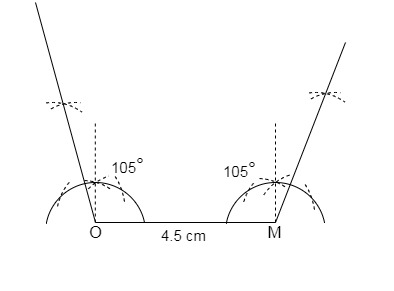Step 3: Open an arc of 6 cm then cut ‘OM’ of 6 cm, as shown in the figure below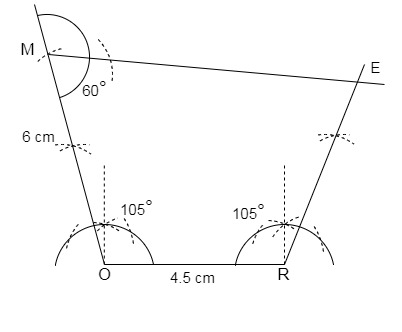Step 4: Now make an angle of 60° from point ‘M’ which will further join point ‘E’.

Step 5: We have the quadrilateral ‘MORE’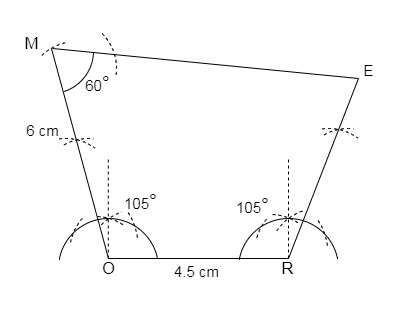Given

PL = 4 cm, LA = 6.5 cm, ∠P = 90°, ∠A = 110°, ∠N = 85°

First we will find angle L,
Sum of angles of a quadrilateral = 360°
∠P + ∠A + ∠N + ∠L = 360°
90° + 110° + 85° + ∠L = 360°
∠L = 360° – 285°
∠L = 75°

Steps for Construction

Step 1: Draw a line segment LA = 6.5 cm.

Step 2: Draw an angle of 75° from point L.

Step 3: Draw another angle of 110° from point A

Step 4: From point L cut PL=4 cm.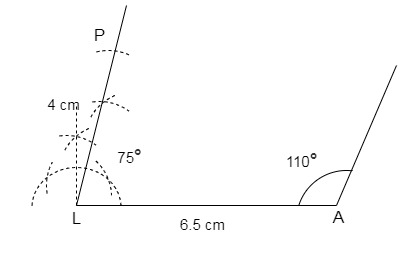Step 5: From point P draw an angle of 90° which intersect the angle line extended through A at N.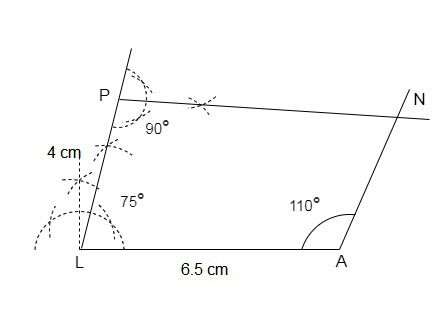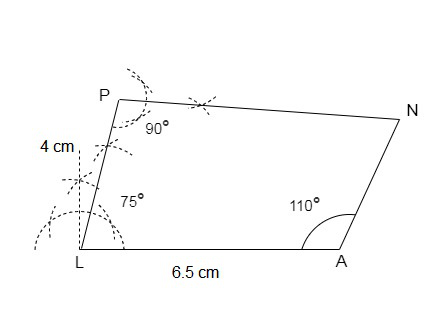Hence, we have our required quadrilateral PLAN.

### 3. Parallelogram HEAR

Given

HE = 5 cm, EA = 6 cm, ∠R = 85°

Steps for Construction

Step 1: Draw a line segment HE = 5 cm

Step 2: From point E draw an angle of 85°.

Step 3: From point E cut EA = 6 cm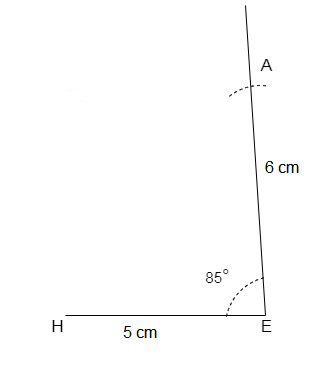Step 4: Draw an arc from center A of 5 cm, draw another arc of 6 cm with centre E, and further mark point R at the intersection of both the arcs. as shown below: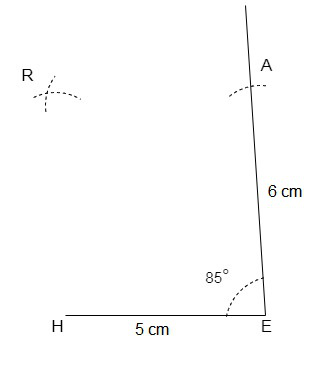Step 5: Join HR and AR respectively.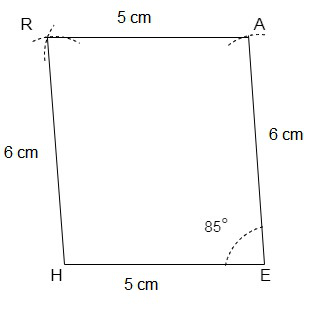Thus, we have the required quadrilateral HEAR.

### 4. Rectangle OKAY

Given

OK = 7 cm, KA = 5 cm

All the angles of a rectangle are 90° and the opposite sides are equal.

Steps for Construction

Step 1: Draw a line segment OK = 7 cm.

Step 2: Draw an 90° angle from point O.

Step 3: Taking point O as radius draw an arc of 5 cm as shown, and mark the point Y.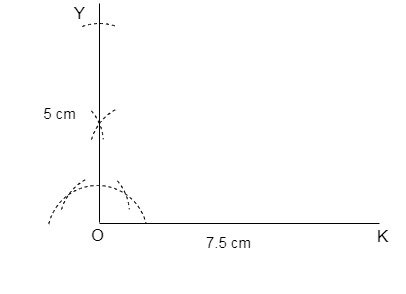Step 4: Taking Points K and Y as centre draw arcs of 5 cm and 7 cm respectively, intersect both the arcs at point A.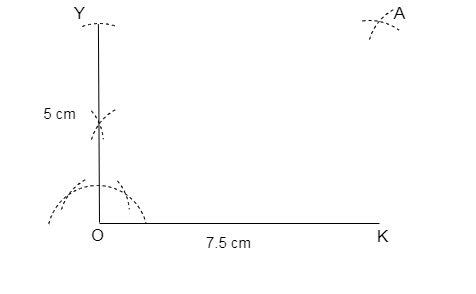Step 5: Join KA and AY.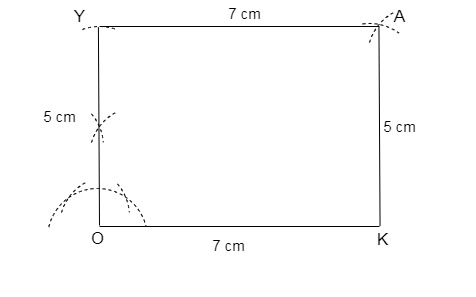Thus, we have the required rectangle OKAY.

My Personal Notes arrow_drop_up